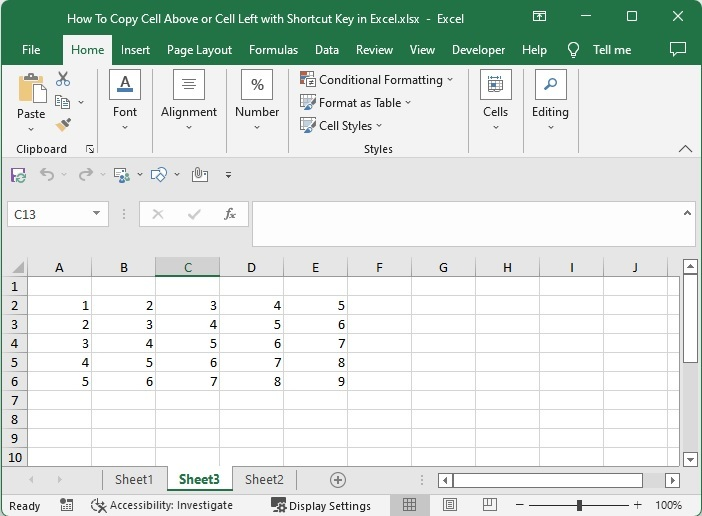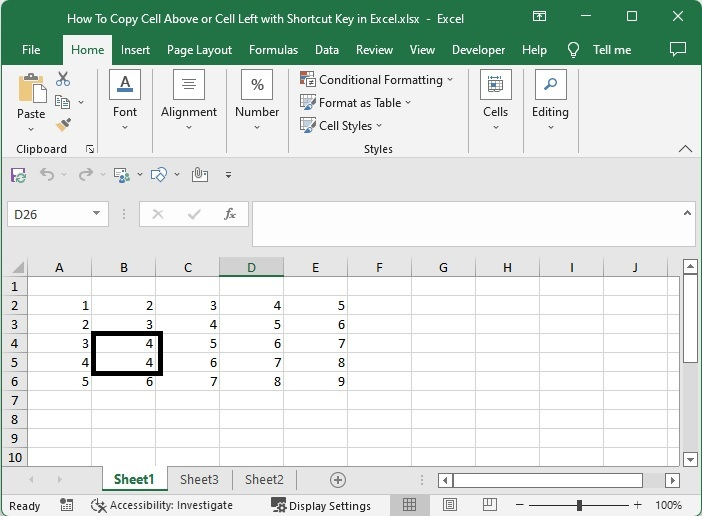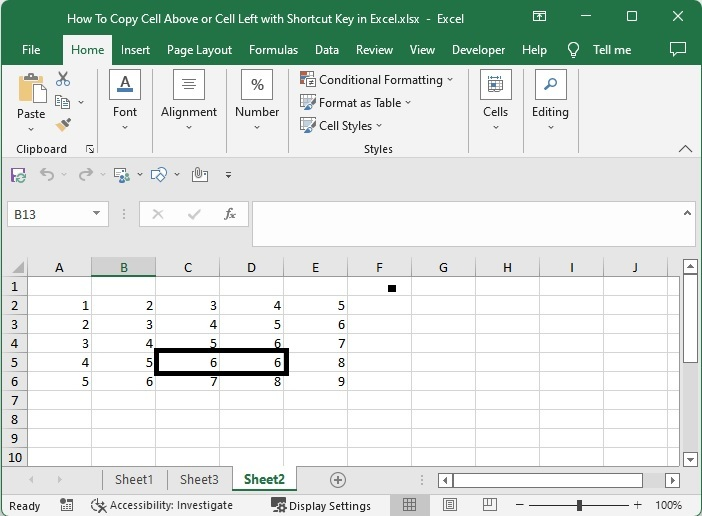# How to Copy Cell Above or Cell Left with Shortcut Key in Excel?

Generally, in Excel, copying the data can be a time-consuming process as it involves many steps like clicking on the cell and using the copy command, then going to the destination cell and using the paste command. To get data from the left or above the current, we can use the simple trick described in this tutorial.

Read this tutorial to learn how you can copy cells above or below with a shortcut key in Excel. Any combination of keys that can complete a task in less time is known as a shortcut key. A shortcut key can help us reduce our time working on the method. We will learn about two shortcut keys that are used to copy the cells in Excel.

## Copy Cell Above with Shortcut Key

Here, we will first click on the cell below that you want to copy and use the command to copy the cell. Let's go over a simple procedure for copying the cell above in Excel using a shortcut key.

### Step 1

Consider an excel sheet containing data in the form of a matrix, as shown in the image below.Now click on the cell that is below the cell you want to copy and use the command CTRL + D, and the value will be copied to that cell. For example, if we need to copy values from cell B4 to cell B5, we would use the command in cell B5, and the result would look like the image below.## Copy Cell Left with Shortcut Key

Here we will use the command directly to copy the data in the left cell. Let's go over a simple procedure for copying left in Excel using a shortcut key.

### Step 1

Let us consider the same data that we used in the above example.

Now, to copy the cell, right-click on it and press CTRL + R. For example, if we need to copy values from cell C5 to cell D5, use the command in cell C5, and our result will be similar to the below image.## Conclusion

In this tutorial, we used simple examples to demonstrate how we can copy cells above or left with a shortcut key in Excel.

Updated on: 07-Mar-2023

512 Views